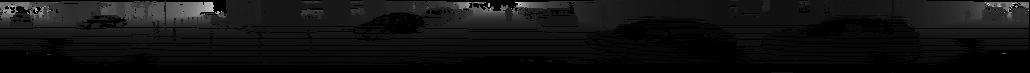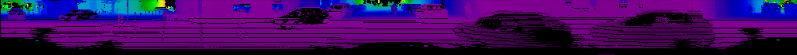## Point Cloud to 360 Degree Panorama

April 3, 2017, 6:28 p.m.

## Summary

A few days ago I created a function that creates 360 degree panoramas from point cloud data. The problem with that previous implementation was that it relied on matplotlib to create the images. Today I created a pure numpy solution, which should make it much faster, and more useful to be used as a preprocessing step.

Here is the function:

```# ==============================================================================
#                                                                   SCALE_TO_255
# ==============================================================================
def scale_to_255(a, min, max, dtype=np.uint8):
""" Scales an array of values from specified min, max range to 0-255
Optionally specify the data type of the output (default is uint8)
"""
return (((a - min) / float(max - min)) * 255).astype(dtype)

# ==============================================================================
#                                                        POINT_CLOUD_TO_PANORAMA
# ==============================================================================
def point_cloud_to_panorama(points,
v_res=0.42,
h_res = 0.35,
v_fov = (-24.9, 2.0),
d_range = (0,100),
y_fudge=3
):
""" Takes point cloud data as input and creates a 360 degree panoramic
image, returned as a numpy array.

Args:
points: (np array)
The numpy array containing the point cloud. .
The shape should be at least Nx3 (allowing for more columns)
- Where N is the number of points, and
- each point is specified by at least 3 values (x, y, z)
v_res: (float)
vertical angular resolution in degrees. This will influence the
height of the output image.
h_res: (float)
horizontal angular resolution in degrees. This will influence
the width of the output image.
v_fov: (tuple of two floats)
Field of view in degrees (-min_negative_angle, max_positive_angle)
d_range: (tuple of two floats) (default = (0,100))
Used for clipping distance values to be within a min and max range.
y_fudge: (float)
A hacky fudge factor to use if the theoretical calculations of
vertical image height do not match the actual data.
Returns:
A numpy array representing a 360 degree panoramic image of the point
cloud.
"""
# Projecting to 2D
x_points = points[:, 0]
y_points = points[:, 1]
z_points = points[:, 2]
r_points = points[:, 3]
d_points = np.sqrt(x_points ** 2 + y_points ** 2)  # map distance relative to origin
#d_points = np.sqrt(x_points**2 + y_points**2 + z_points**2) # abs distance

# We use map distance, because otherwise it would not project onto a cylinder,
# instead, it would map onto a segment of slice of a sphere.

# RESOLUTION AND FIELD OF VIEW SETTINGS
v_fov_total = -v_fov + v_fov

v_res_rad = v_res * (np.pi / 180)
h_res_rad = h_res * (np.pi / 180)

# MAPPING TO CYLINDER
x_img = np.arctan2(y_points, x_points) / h_res_rad
y_img = -(np.arctan2(z_points, d_points) / v_res_rad)

# THEORETICAL MAX HEIGHT FOR IMAGE
d_plane = (v_fov_total/v_res) / (v_fov_total* (np.pi / 180))
h_below = d_plane * np.tan(-v_fov* (np.pi / 180))
h_above = d_plane * np.tan(v_fov * (np.pi / 180))
y_max = int(np.ceil(h_below+h_above + y_fudge))

# SHIFT COORDINATES TO MAKE 0,0 THE MINIMUM
x_min = -360.0 / h_res / 2
x_img = np.trunc(-x_img - x_min).astype(np.int32)
x_max = int(np.ceil(360.0 / h_res))

y_min = -((v_fov / v_res) + y_fudge)
y_img = np.trunc(y_img - y_min).astype(np.int32)

# CLIP DISTANCES
d_points = np.clip(d_points, a_min=d_range, a_max=d_range)

# CONVERT TO IMAGE ARRAY
img = np.zeros([y_max + 1, x_max + 1], dtype=np.uint8)
img[y_img, x_img] = scale_to_255(d_points, min=d_range, max=d_range)

return img
```

And here is an example of it being used, using values configured for the Velodyne HDL 64E.

```im = point_cloud_to_panorama(points,
v_res=0.42,
h_res=0.35,
v_fov=(-24.9, 2.0),
y_fudge=3,
d_range=(0,100))
```

And here is an example of the output when used on a frame from the Kitti dataset.And the same image rendered using a spectral colormapping:One thing i am not entirely happy about is that the images are not turning out as nicely as in the matplotlib version. The images are not as smooth. There horizontal streaks of empty values. Im sure this has something to do with the way discretization of the point positions to pixel positions was done.

## Next Steps

The next steps will be to create the images with multiple channels representing different things. Currently the image only encodes depth. This should be easy but i just didnt get time to do it today.